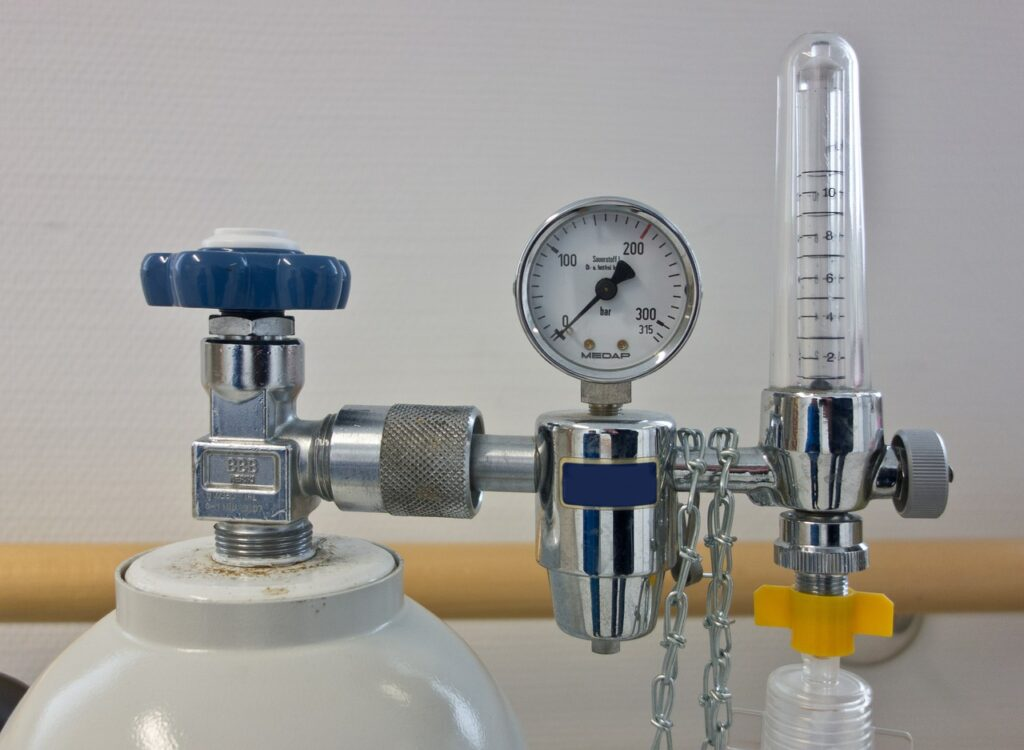# Pressure in O Level Physics – What’s tested?Let’s look at what’s tested on the topic pressure in O Level Physics.

In this chapter, students are expected to do some calculations, so do remember the different formulae for pressure. I’ve compiled a list of the formulae tested in O Level Physics here. In addition, students are expected to know the definition of pressure. Apart from that, there’s also some common explanation questions in this topic.

Check out our video here to watch what’s tested in this chapter.

Use our checklist below to check whether you know the following concepts after revision.

## Checklist for Pressure as tested in O Level Physics

define pressure

apply the formula pressure = force/ area

how a hydraulic press works

pressure in liquid = height x density x gravitational field strength (P = hρg)

Using height of liquids to measure atmospheric pressure e.g. using a barometer

Use of manometer to measure gas pressure